# ILP Part 6 – Faster multiplication

This is the sixth part of the ILP series. For your convenience you can find other parts in the table of contents in Part 1 – Boolean algebra

Last time we saw how to define minimum in ILP. We also know how to multiply variables using long multiplication algorithm. Our algorithms work, however, they are slow. Today we are going to utilizefunction to speed up multiplication.

# General idea

We know how to multiply variables. Basically, we decompose both arguments and extract digits. Next, we multiply every pair of digits using conjuction, and multiply the result by power of two (which is constant).
As we said in part 2, this requires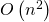temporary variables because we need to store every conjunction in some temporary variable. However, when we perform long multiplication on a piece of paper we do not multiply every two digits but we multiply digit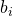by whole argument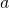(using notation from part 2). We can do the same in ILP.

## Improvement

We know that we cannot multiply variables just like that because we need to use only linear constraints so once again we are going to use some trick. We have the following variables: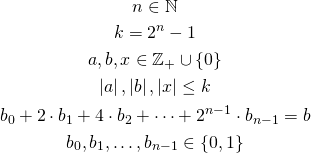We have arguments (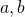), result (), decomposition (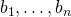), and the maximum possible value (). Now we are going to multiply every digitby argumentusingfunction.

Let’s consider the value of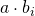. Whenis zero then the whole value is zero (because it is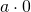). Whenis one the result is equal to. We also know that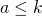which implies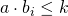. This is in fact equivalent to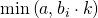. Whenis zero we get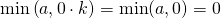. Whenis one we obtain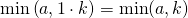and becausewe get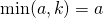. We also need to remember thatis a constant value so we are allowed to calculate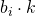.

## Final formula

Now we can define the final formula: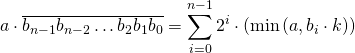We simply multiply every digit byand then by the power of two.

# Summary

We are now able to calculate multiplication using onlytemporary variables which is a great improvement. In the next part we are going to see how to multiply negative values using today’s algorithm and absolute value function defined in previous part.